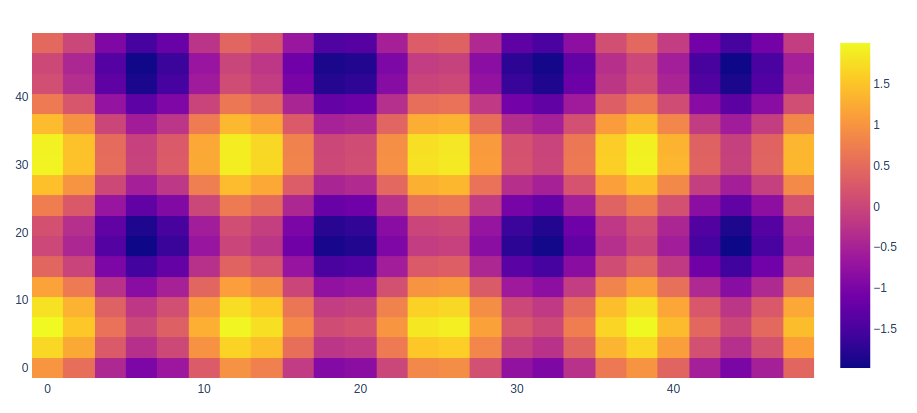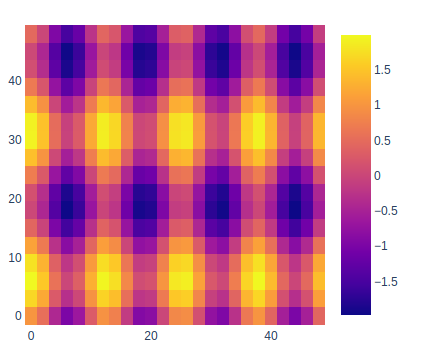# Create Heatmaps using graph_objects class in Plotly

A Plotly is a Python library that is used to design graphs, especially interactive graphs. It can plot various graphs and charts like histogram, barplot, boxplot, spreadplot, and many more. It is mainly used in data analysis as well as financial analysis. plotly is an interactive visualization library.

## Heatmaps using graph_objects

A heatmap is a two-dimensional graphical representation of data where the individual values that are contained in a matrix are represented as colors.

Syntax: plotly.graph_objects.Heatmap(arg=None, autocolorscale=None, colorbar=None, colorscale=None, x=None, y=None, z=None, **kwargs)

Parameters:

arg: dict of properties compatible with this constructor

autocolorscale: Determines whether the colorscale is a default palette (autocolorscale: true) or the palette determined by colorscale.

colorbar: plotly.graph_objects.heatmap.ColorBar instance or dict with compatible properties

colorscale: Sets the colorscale. The colorscale must be an array containing arrays mapping a normalized value to an rgb, rgba, hex, hsl, hsv, or named color string.

x: Sets the x coordinates.

y: Sets the y coordinates.

z: Sets the z coordinates.

Example:

## Python3

 `import` `plotly.graph_objects as go ` ` `  `feature_x ``=` `np.arange(``0``, ``50``, ``2``)  ` `feature_y ``=` `np.arange(``0``, ``50``, ``3``)  ` ` `  `# Creating 2-D grid of features  ` `[X, Y] ``=` `np.meshgrid(feature_x, feature_y)  ` ` `  `Z ``=` `np.cos(X ``/` `2``) ``+` `np.sin(Y ``/` `4``)  ` ` `  `fig ``=` `go.Figure(data ``=` `     ``go.Heatmap(x ``=` `feature_x, y ``=` `feature_y, z ``=` `Z,)) ` ` `  `fig.show()`

Output:## Presenting Heat map with Unequal Block Sizes

In plotly, it is possible to show the unequal sizes of the blocks as to make the data differentiate between each other to make it more clear while presenting as the data has different height and width compared to each other.

Example:

## Python3

 `import` `plotly.graph_objects as go ` ` `  `feature_x ``=` `np.arange(``0``, ``50``, ``2``) ` `feature_y ``=` `np.arange(``0``, ``50``, ``3``) ` ` `  `# Creating 2-D grid of features ` `[X, Y] ``=` `np.meshgrid(feature_x, feature_y) ` ` `  `Z ``=` `np.cos(X ``/` `2``) ``+` `np.sin(Y ``/` `4``) ` ` `  `fig ``=` `go.Figure(data``=``go.Heatmap( ` `  ``x``=``feature_x, y``=``feature_y, z``=``Z,)) ` ` `  `fig.update_layout( ` `    ``margin``=``dict``(t``=``200``, r``=``200``, b``=``200``, l``=``200``), ` `    ``showlegend``=``False``, ` `    ``width``=``700``, height``=``700``, ` `    ``autosize``=``False``) ` ` `  ` `  `fig.show() `

Output:Whether you're preparing for your first job interview or aiming to upskill in this ever-evolving tech landscape, GeeksforGeeks Courses are your key to success. We provide top-quality content at affordable prices, all geared towards accelerating your growth in a time-bound manner. Join the millions we've already empowered, and we're here to do the same for you. Don't miss out - check it out now!

Previous
Next